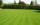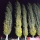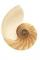# Equation with fractions

Solve equation:It is equation with fractions.

Result

x =  -14

#### Solution:

1-(2x-5)/6 =3-x/4

x = -14

x = -14

Calculated by our simple equation calculator.

Leave us a comment of example and its solution (i.e. if it is still somewhat unclear...):

Showing 0 comments:Be the first to comment!#### To solve this example are needed these knowledge from mathematics:

Need help calculate sum, simplify or multiply fractions? Try our fraction calculator. Do you have a linear equation or system of equations and looking for its solution? Or do you have quadratic equation?

## Next similar examples:

1. Simple equation 6Solve equation with one variable: X/2+X/3+X/4=X+4
2. Equation with xSolve the following equation: 2x- (8x + 1) - (x + 2) / 5 = 9
3. EqnSolve equation with fractions: 2x/3-50=40+x/4
4. Sales storesThe first sales store passed the 1/3 and the second 2/5 of the total amount of goods.In third store passed the 2/3 rest of the goods. The remaining 40 kg of goods put into fourth store. How many kilograms of goods pass to a third store?
5. Mr. HappyMr. Happy planted 36.6 meters square gardens grass; It's a third of the garden more than half of the garden. What is square area of this garden?
6. Unknown numberI think the number - its sixth is 3 smaller than its third.
7. Cleaning windowsCleaning company has to wash all the windows of the school. The first day washes one-sixth of the windows of the school, the next day three more windows than the first day and the remaining 18 windows washes on the third day. Calculate how many windows ha
8. FractionsThree-quarters of an unknown number are 4/5. What is 5/6 of this unknown number?
9. PearsThere were pears in the basket, I took two-fifths of them, and left six in the basket. How many pears did I take?
10. UN 1If we add to an unknown number his quarter, we get 210. Identify unknown number.
11. PoplarHow tall is a poplar by the river, if we know that 1/5 of its total height is a trunk, 1/10th of the height is the root and 35m from the trunk to the top of the poplar?
12. Class 8.AThree quarters of class 8.A went skiing. Of those who remained at home one third was ill and the remaining six were on math olympic. How many students have class 8.A?
13. Determine the numberDetermine the number x that ?.
14. SeamstressThe seamstress cut the fabric into 3 parts. The first part was the eighth fabric, the second part was three-fifths of the fabric and the third part had a length of 66 cm. Calculate the original length of the fabric.
15. Simple equationSolve for x: 3(x + 2) = x - 18
16. EquationSolve the equation: 1/2-2/8 = 1/10; Write the result as a decimal number.
17. Unknown numberIdentify unknown number which 1/5 is 40 greater than one tenth of that number.